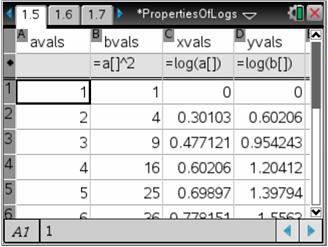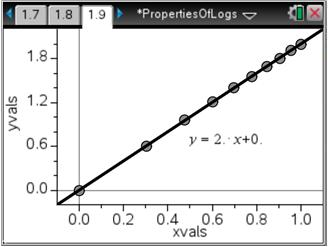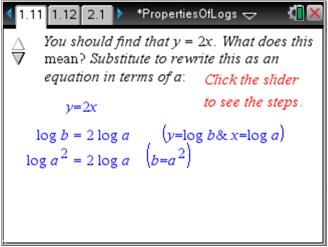# Activities

••• ##### Subject Area

• Math: Algebra II: Logarithms and Exponentials

• ##### Author9-12

75 Minutes

• ##### Device
•TI-Nspire™ CX/CX II
•TI-Nspire™ CX CAS/CX II CAS
• TI-Nspire™ Navigator™
• TI-Nspire™
• TI-Nspire™ CAS
• ##### Software

TI-Nspire™
TI-Nspire™ CAS

3.2

• ##### Other Materials
By Lynne Plettenberg

## Properties of Logarithms

#### Activity Overview

Students use a combination of algebra and graphing to discover the properties of logarithms. Problems 1 and 2 cover the Power and Product Properties of logarithms, respectively; Problem 3 covers the Quotient Property in addition to introducing log-log "paper", a grid with a logarithmic scale on each axis.

#### Key Steps

•Students are introduced to the properties of logarithms. They will use the properties to answer the question.
How can you simplify the logarithm of a power?
Using a spreadsheet, students calculate the value of b = a2 from the values of a.  Then, students will graph the data from Columns A and B as a scatter plot and observe the resulting shape.

•Students will calculate the values of x = log(a) and y = log(b) and then graph them in a scatter plot on page 1.9.

•Students will view how to rewrite the logarithmic equation. They will see the Power Property of Logarithms revealed.